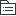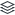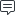# 中国古代历史

373
|
100

## 相关模板推荐

•## 中国古代历史 —— 作品大纲

•公元前157年
•公元前180年
• 措施
• 轻徭薄赋
• 提倡以农为本，关心农桑
• 十五税一改三十税一
• 服役一年一次改为三年一次
• 减轻刑罚
• 重视“以德化民”
• 废除一些严刑苛法
• 提倡节俭
• 提倡勤俭治国
• 皇帝以身作则
• 结果
• 政治清平
• 经济发展
• 国力增强
•文景之治
•公元前202年
• 休养生息
• 原因：
• 巩固政权和稳定社会局势
• 汉高祖吸取秦朝暴政速亡的教训
• 措施：
• 解甲归田：下令“兵皆罢归家”，让士兵还家务农
• 释奴为民：将奴婢释放为平民，以增加农业劳动力
• 轻徭薄赋
• 减轻田租，定税率为十五税一
• 相应地减免徭役及兵役
• 影响：汉初的经济逐渐得以恢复和发展，社会局势得以稳定
• 西汉建立
• 皇帝：汉高祖刘邦
• 都城：长安（陕西西安）
• 现状：
• 社会生产遭到严重的破坏，国家贫困，经济萧条
• 原因：秦朝的残暴统治和秦末的战乱（秦末农民战争、楚汉之争）
•初汉
•公元前206年
• 楚汉相争
• 时间
• 四年
• 人物
• 项羽和刘邦
• 目的
• 争夺帝位
• 结果
• 刘邦胜利
• 原因
• 项羽刚愎自负，一味依赖武力，失去民心
• 刘邦注重收揽民心，善于用人
• 得民心者得天下，失民心者失天下
•公元前207年
• 秦朝灭亡
• 人物
• 刘邦
• 结果
• 秦朝灭亡
• 成语
• 约法三章
• 巨鹿之战
• 人物
• 项羽
• 结果
• 以少胜多，大败秦军主力
• 成语
• 破釜沉舟
•公元前209年
• 陈胜吴广起义
• 地点
• 大泽乡
• 原因
• 遇雨误期，按律当斩
• 秦的暴政
• 口号
• 王侯将相，宁有种乎
• 方式
• 农民揭竿而起
• 经过
• 陈胜称王，在陈建立“张楚”政权
• 向西进攻，直逼咸阳
• 结果
• 失败
• 意义
• 中国历史上第一次大规模的农民起义
• 鼓舞后世劳动人民反抗残暴的统治
•秦末
•公元前221年
• 秦朝暴政
• 政策
• 沉重的赋税
• 繁重的徭役和兵役
• 刑罚残酷
• 秦二世的统治更加残暴
• 影响
• 社会经济严重破坏，加重了人民的负担和苦难，激起人民的反抗
• 秦朝政策
• 措施
• 政治
• 中央集权制度
• 皇帝
• 太尉
• 管理军事
• 丞相
• 管理政事
• 御史大夫
• 监察百官
• 经济
• 统一货币
• 统一度量衡
• 文化
• 统一文字
• 交通
• 车同轨
• 开灵渠
• 军事
• 北击匈奴修长城
• 影响
• 郡县制沿用了2000多年，对中国历史产生了深远影响
• 促进了各地的经济交流，巩固了国家统一
• 秦朝建立
• 都城
• 咸阳（陕西西安）
• 开国皇帝
• 秦始皇嬴政
• 疆域
• 东至东海，西到陇西，北至长城，南到南海
• 意义
• 结束了春秋战国以来长期争战混战的局面
• 建立起我国历史上第一个统一的多民族的封建国家
•公元前230年
• 秦灭六国
• 顺序
• 韩、赵、魏、楚、燕、齐
• 策略
• 由近及远，各个击破
• 原因
• 人民希望结束战乱，安定生活
• 商鞅变法，秦国强大
• 秦王嬴政雄才大略
•秦初
•公元前475年
• 百家争鸣
• 背景
• 战国时期，旧的社会制度进一步崩溃
• 新的社会制度逐步确立
• 学术思想领域非常活跃
• 不同的学派，各陈其说，史称诸子百家
• 派别
• 墨家
• 代表人
• 墨子
• 主张
• 兼爱非攻
• 提倡节俭
• 启示
• 构建和谐社会，反对侵略战争，勤俭治国
• 儒家
• 孟子
• 主张
• 实行仁政
• 民为贵，社稷次之，君为轻
• 反对一切非正义的战争
• 荀子
• 实行礼治
• 明确尊卑等级
• 道家
• 代表人
• 庄子
• 主张
• 治国要顺其自然和民心
• 追求精神自由
• 保持独立的人格
• 启示
• 积极地面对和看待问题
• 法家
• 代表人
• 韩非
• 主张
• 以法治国；建立中央集权专制统治
• 启示
• 积极改革，勇于创新
• 影响
• 百家争鸣促进了思想和学术的繁荣
• 中国古代第一次思想文化发展的高峰
• 为中国古代文化的发展奠定了基础
• 对后世有十分重要而深远的影响
• 都江堰
• 时间
• 公元前256年
• 地点
• 成都
• 人物
• 李冰
• 构成
• 由渠首和灌溉网两大系统工程构成
• 功能
• 防洪、灌溉、水运
• 影响
• 使成都平原成为沃野，变成天府之国
• 两千多年来，都江堰一直发挥着巨大的作用，在世界水利史上绝无仅有，充分反映出我国人民的智慧
• 商鞅变法
• 背景
• 根本原因
• 战国时期，铁制农具和牛耕的使用进一步推广，社会生产力水平不断提高，新兴地主阶级的势力增强
• 发展需要
• 为适应社会政治经济的变化，各诸侯国统治者实行变法改革，确立新的政治经济秩序，以求富国强兵，在兼并战争中取胜
• 目的
• 富国强兵，在兼并战争中取胜
• 子主题
• 时间
• 公元前356年
• 子主题
• 人物
• 秦孝公任用商鞅主持变法
• 内容
• 政治
• 确立县制，由国君直接派官吏治理（建立县制，对后世影响最为深远）
• 废除贵族的世袭特权（损害了旧贵族的利益，是后来商鞅被处死的原因）
• 改革户籍制度，加强对人民的管理
• 严明法度，禁止私斗
• 经济
• 废除井田制，允许土地买卖（最能体现变法性质）
• 鼓励耕织，生产粮食、布帛多的人可免除徭役（国富）
• 统一度量衡
• 军事
• 奖励军功，对有军功者授予爵位并赏赐土地（兵强）
• 作用
• 使秦国的国力大为增强，提高了军队的战斗力，一跃成为最强盛的诸侯国，为以后秦统一全国奠定了基础
• 兼并战争
• 特点
• 规模很大
• 参战兵力多
• 交战区域广
• 持续时间长
• 战役
• 桂陵之战
• 齐、魏、赵-围魏救赵
• 马陵之战
• 齐、魏、韩-增兵减灶、孙庞
• 长平之战
• 秦胜赵败、杀40万
• 结果
• 魏国、齐国、赵国、秦国先后崛起
• 秦国成为最强盛的诸侯国对东方六国构成威胁
• 影响
• 为加速结束分裂，实现国家统一奠定了基础
• 战国七雄
• 形成
• 三家分晋
• 田氏代齐
• 齐楚秦燕赵魏韩
•东周战国
•公元前770年
• 经济发展
• 农业
• 铁制农具和牛耕出现，促进了农业上的深耕细作
• 手工业
• 规模扩大
• 商业
• 出现商品交换市场、金属货币
• 王室衰微
• 原因
• 周平王东迁洛邑
• 社会动荡，各种制度遭到破坏
• 分封制逐步遭到瓦解
• 表现
• 周王室的统治势力大减，已无力控制诸侯
• 诸侯不再听从王命，各自为政
• 诸侯不再定期向天子纳贡，导致周王室在财政上陷入困境
• 结果
• 周王室地位下降，大的诸侯国势力崛起
• 他们竞相称霸，操控政治局面，实际上取代了周天子的地位
• 诸侯争霸
• 背景
• 政治、经济发展不平衡
• 诸侯国为了自身的利益相互展开争斗
• 民族间的矛盾也有所发展
• 经历
• 齐桓公
• 春秋时期的第一个霸主
• 晋文公
• 楚庄王
• 秦穆公
• 吴国和越国北上争霸
• 卧薪尝胆
• 影响
• 消极
• 给社会和人民带来巨大的灾难
• 积极
• 在争霸过程中，小的诸侯国被灭掉
• 强大诸侯国的疆域扩展，加快统一步伐
• 出现了大规模的民族交融
• 主要思想
• 道家
• 创始人
• 老子
• 主要思想
• 顺应自然
• 事物都有其对立面，对立的双方可以相互转化
• 主张无为而治
• 启示
• 辩证地看待问题
• 儒家
• 创始人
• 孔子
• 政治思想
• 核心思想是“仁”
• 主张以德治国
• 主张实行德政
• 教育思想
• 创办私学，主张“有教无类”
• 注重道德教育和文化知识教育
• 发现和总结出许多教学原则和方法
• 因材施教
• 温故知新
• 著作
• 《论语》
• 文化成就
• 传承中国古代文化经典和学术思想
• 启示
• 以人为本
• 构建和谐社会
• 尊重教育教学规律
• 采取科学的学习方法
• 兵家
• 创始人
• 孙武
• 主张
• 知彼知己，百战不殆
• 著作
• 《孙子兵法》
•东周春秋
•公元前1046年
• 建立者
• 周武王
• 都城
• 镐京
• 分封制
• 目的
• 稳定政治形势
• 巩固疆土
• 内容
• 依据
• 血缘关系远近
• 功劳大小
• 受封人
• 宗亲
• 功臣
• 权力
• 管理土地和人民的权力
• 可以在封地内再分封
• 义务
• 向周王进纳贡物
• 服从周王调兵
• 作用
• 保证了周王朝对地方的控制
• 稳定了政局
• 扩大了统治范围
• 实质
• 确立了周王朝的社会等级制度
• 周天子 → 诸侯 → 卿大夫 → 士
• 弊端
• 诸侯国具有较大的独立性
• 当诸侯国力量强大后，会威胁到周天子的统治地位
• 最终导致春秋战国的战乱与纷争
• 亡国之君
• 周幽王，烽火戏诸侯
• 特色
• 奴隶社会的鼎盛时期
•公元前1600年
• 青铜器
• 用途
• 饮食
• 祭祀
• 军事
• 功能
• 食器
• 礼器
• 特点
• 种类丰富
• 数量众多
• 制作工艺高超
• 代表
• 司母戊鼎
• 迄今世界上出土的最大青铜器
• 四羊方尊
• 造型奇特
• 利簋
• 制作工艺
• 成分
• 铜锡
• 方法
• 泥范铸造法
• 甲骨文
• 发现情况
• 时间
• 1899年
• 人物
• 清朝王懿荣
• 含义
• 刻写在龟甲和牛、羊等兽骨上的文字
• 记载内容
• 祭祀、战争、农牧业、管制、刑法、医药、天文历法
• 发现意义
• 甲骨文是中国发现的古代文字年代最早、体系较为完整的文字
• 对中国文字的形成与发展有深远的影响
• 证明我国有文字可考的历史从商朝开始
• 甲骨文已经具备了汉字的基本结构，是汉字形成与发展的重要阶段
• 造字特点
• 象形
• 指事
• 会意
• 形声
• 建立者
• 商汤
• 都城
• 盘庚迁都至殷
• 亡国之君
• 纣王
• 武王伐纣
• 牧野大战，商朝灭亡
•公元前2070年
• 建立者
• 大禹
• 都城
• 阳城
• 制度
• 中国第一个奴隶制国家
• 禅让制改为世袭制（启）
• 亡国之君
• 桀（桀骜不驯）
• 特色
• 盘庚 迁都至殷，统治稳定
•奴隶社会
•距今5000年
• 尧、舜、禹
• 禅让制
• 将部落首领位子传给贤德之人
• 大禹治水
• 与群众同甘共苦，三过家门而不入，治水有功
• 体现了刻苦耐劳，坚持不懈，大公无私的精神
• 炎黄
• 涿鹿之战
• 炎黄部落打败蚩尤部落
• 阪泉之战
• 黄帝打败炎帝，炎黄联盟形成
• 影响
• 华夏族逐渐形成
• 炎帝、黄帝被尊崇为中华民族的人文始祖
• 贡献
• 炎帝
• 教民开垦耕种，制作生产工具，种植五谷和蔬菜
• 制作乐器琴瑟，有最早的图文和历法知识
• 制作陶器，发明纺织，会煮盐，教人们通商交换
• 黄帝
• 建造宫室
• 制作衣裳
• 挖掘水井
• 制造船只
• 教人炼銅
• 弓箭和指南车
• 其他
• 仓颉创造文字
• 伶伦制作音律
• 隶首发明算盘
• 嫘祖擅长纺织并会缫丝
•距今6000年
• 半坡人
• 居住地
• 黄河流域的陕西西安
• 气候干旱寒冷，风沙大
• 房屋样式
• 半地穴式圆形房屋
• 抵风挡雨
• 保暖
• 生产工具
• 使用磨制石器、制作骨器、角器
• 农业
• 种植水稻
• 原始畜牧业
• 饲养猪、狗；打猎捕鱼
• 手工业
• 制作彩陶和乐器；会纺织、制衣
•距今7000年
• 河姆渡人
• 居住地
• 长江流域的浙江余姚
• 潮湿温热
• 房屋样式
• 干栏式房屋
• 通风防潮
• 防蛇虫之害
• 生产工具
• 使用磨制石器，如骨耜
• 农业
• 种植粟
• 畜牧业
• 饲养猪、狗和水牛
• 手工业
• 制造陶器、玉器和乐器
• 会雕刻，懂得使用天然漆
•原始社会后期
•距今3万年
• 山顶洞人
• 发现地点
• 北京周口店龙骨山顶部
• 体貌特征
• 已经具有现代人类的特征
• 生产工具
• 磨已掌握钻孔光技术和磨制技术（骨针）
• 观念
• 已经有了审美的观念
•距今20-70万年
• 北京人
• 发现地点
• 北京西南周口店龙骨山上
• 体貌特征
• 上肢与现代人相似，能够直立行走，保留了猿的某些特征
• 生产生活
• 使用打制石器、木棒
• 采集、狩猎
• 会使用天然火，会保存火种
• 社会组织
• 过群居生活，原始社会
• 意义
• 北京人是世界上最重要的原始人类之一
• 迄今所知世界上内涵最丰富、材料最齐全的直立人遗址
•距今170万年
• 元谋人
• 发现地点
• 云南元谋乡
• 生活情况
• 能制造工具，知道用火
• 意义
• 我国境内目前确认的最早古人类
•原始人类